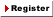New User RegisterLogin Member: Email: Password:
Math
 Subjects: Arithmatic(11)
 OverView : This category covers Quantity : Natural numbers, Integers Space : Geometry, Trigonometry Change: Calculus, Differential equations, Dynamical systems Foundations : Set theory, Cryptography, Graph theory Applied mathematics : Probability, Statistic, Financial mathematics, Game theory, Operations research